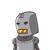# How will you show that (17 x 11 x 2) + (17 x 11x 5) is a composite number.​

How will you show that (17 x 11 x 2) + (17 x 11x 5) is a composite number.​

### 1 thought on “How will you show that (17 x 11 x 2) + (17 x 11x 5) is a composite number.​”

1.Step-by-step explanation:

we can write is as ,

(17×11×2+17×11×5)

17×11(1×1×2+1×1×5)  {17 and 11 common }

17×11(2+5)

17×11×7

=1309

1309 is a composite number